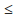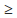# TARGET SBI EXAM 2018: REASONING – MOT-2

Dear Bankersdaily Aspirants,

Hope , Your preparation for SBI Clerk & PO exam 2018 is good . Since SBI Clerk & PO exam is a Two level which will be conducted in an online mode, Competition will be higher for this SBI Clerk & PO Exam and the most important thing , Current affairs section will be there in this exam. So aspirants have to concentrate in Four sections namely Aptitude, Reasoning, English, Current Affairs.

The person with high speed and very good accuracy only can crack this exam to taste the success. The candidates will be selected on the basis of performance in this online exam test. So the candidate with consistent hard work and regular practice will crack the exam very easily.

Exam: SBI Clerk & PO Exam 2018

Topic: MOT-2

Timing: 7 minutes

D.1-5) In the following questions, the symbols @, %, #, \$ and * are used with different meanings explained below. Read the statements carefully and answer the following questions:

P @ Q means P is neither smaller than nor equal to Q.

P % Q means P is neither greater than nor equal to Q.

P * Q means P is neither greater than nor smaller than Q.

P # Q means P is not greater than Q.

P \$ Q means P is not smaller than Q.

1. If the following conclusions O % B and C \$ E are definitely true, then which of following statement is logically satisfies the conclusions?

a) E \$ P @ B* N @ C % O *Y

b) O % Y % N @ B % C \$ P * E

c) Y \$ O % N * B # C \$ P * E

d) E * O * Y % N @ C @ B *P

e) None of these.

2. If the following conclusions A % E and D @ G are should always true, then which of the following statement is logically satisfies the conclusions?

a) A # C \$ D # E # F * G % H

b) H * C * E % F # D @ A % G

c) E @ C * F % H @ A % D * G

d) A # C * F % E \$ D * H @ G

e) None of these.

3. If the following conclusions Z % Y and W # V should always true, then which of the following statement is logically satisfies the conclusions?

a) None of these.

b) Z \$ K # R * I # W % Y @ U * V

c) W % Z % R * U % K \$ I *V % Y

d) V * Z % U @ R % W \$ I * K @ Y

e) K @ Z % R * Y \$ W # I * V @ U

4. In the following conclusions T # S should be false and S \$ P should be true. Then which of the following statement is logically satisfies the conclusions?

a) S @ O % P * Q #G \$ R @ T # V

b) P \$ O % T * Q \$ V \$ R % H * S

c) R* O# S @ G # Q # V @ T * P

d) G @ O % T * Q \$ S \$ R * P # V

e) None of these.

5. In the following conclusions, C @ N should be false and N \$ G should be true. Then which of the following statement is logically satisfies the conclusions?

a) N % A % L * C \$ D @ G * R # M

b) C @ L * A @ G \$ D * M @ R * N

c) C @ L @ A % N \$ D \$ G * R # M

d) C * L @ A % G \$ D % R @ M @ N

e) None of these

D.6-10) In the following questions, the symbols @, &, #, \$, * are used with different meanings explained below. Study the following information to answer the given Questions:

A\$B” means “A is not smaller than B”

A@B” means “A is neither smaller than nor equal to B”

A#B” means “A is neither greater than nor equal to B”

A&B” means “A is neither greater than nor smaller than B”

A*B” means “A is not greater than B”

6. Statements: Q \$ J, E@ D, G # E, Q\$D

Conclusions:

I. G # D

II. Q @ E

III. D * Q

IV. J # E

a) Only I is true

b) None is true

c) Only III is true

d) Only II is true

e) Only IV is true

7. Statements: A * B, C * D, B @ E, A @ C

Conclusions:

I. B @ C

II. A \$ B

III. A @ B

IV. A @ E

a) Only II is true

b) None is true

c) Only III and IV are true

d) Only I is true

e) Only I and IV are true

8. Statements:

E * D, M # O, D \$ M, O @ P

Conclusions:

I. P # M

II. D @ O

III. E # O

IV. D @ P

a) Only II is true

b) None is true

c) Only III is true

d) Only I is true

e) Only IV is true

9. Statements:

Z @ Y, Y # X, X & F, F * W

Conclusions:

I. W \$ X

II. F @ Y

III. Z @ W

IV. Y @ W

a) Only I, II and III are true

b) Only I and II are true

c) Only I and III are true

d) Only I, III and IV are true

e) All are true

10. Statements:

C # D, D * E, E @ F, F \$ G

Conclusions:

I. G # D

II. G @ E

III. D # F

IV. E # C

a) Only II is true

b) Only IV is true

c) Only III is true

d) Only I is true

e) None is true

D.1-5)

 @ > # ≤ % < \$ ≥ * =

1. (c)

Y ≥ O < N = BCP= E

2. (d)

A ≤ C = F < E ≥ D = H > G

3. (e)

K > Z < R = Y ≥ W ≤ I = V > U

4. (d)

G > O < T = Q ≥ S ≥ R = PV

5. (c)

C > L > A < N ≥ D ≥ G = RM

D.6-10)

 \$ ≥ # < * ≤ @ > & =

6. (c)

G < E > D ≤ Q ≥ J

7. (c)

D ≥ C < A ≤ B > E

8. (b)

E ≤ D ≥ M < O > P

9. (b)

Z > Y < X = F ≤ W

10. (e)

C < D ≤ E > F ≥ G

For the other Sections in the Day 3 of the TARGET SBI PLANNER , please check the below given links.

Aspirants can also check the other topics from the SBI CLERK STUDY PLANNER from the link given below. DO bookmark the given link as all the posts will be updated in that page and aspirants can check the daily updates from this page or from the Homepage of Bankersdaily.